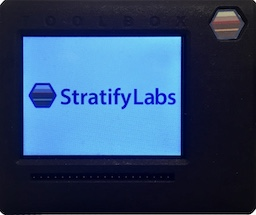# Sensing the Source Current in an Embedded Application###### Introducing the Stratify Toolbox

Are you a printf()-er or a code-stepper? The Stratify Toolbox is a printf()-ing awesome debug tool!This is a simple, low-cost way to measure the current output at the power source of an embedded system using a microcontroller’s ADC input.

The above sensor circuit measures the current at the power source of an embedded system. The power source shown is a battery but can be any DC supply source. The above schematic shows VCC as both the battery voltage and the op-amp voltage. However, the battery voltage can be any value, and the circuit will still work. The key components of the circuit are the sensor resistor (RSENSE) and the amplifier (U1). The voltage input to U1 (the negative terminal of the battery) will always be a negative voltage with respect to ground. For this reason, U1 is configured as an inverting amplifier where:

$$V_O = -V_I \cdot \frac{R_1}{R_2}$$

## Analog and Digital Filtering

The output of the amplifier is then passed through a low pass filter (LPF) consisting of R3 and C1. The filter has a cutoff frequency of:

$$\omega_c = \frac{1}{2 \pi R_3C_1}$$

The purpose of the filter is to decrease the amount of noise that is measured at the ADC input. For best results, the microcontroller should sample the ADC input at double the cutoff frequency of the low pass filter (read more about the Nyquist frequency). If the signal is still too noisy, digital filtering can be used to smooth it out after it is sampled. An effective digital filter, that is easy-to-implement on resource constrained systems, is represented by the following equation which calculates an exponential moving average (this is a type of infinite impulse response, or IIR, LPF). The “x” value is read from the microcontroller ADC, the “y” value is the average, and alpha is a constant between zero (infinite averaging) and one (no averaging).

$$y[n] = x[n] \alpha + y[n-1] \cdot (1 - \alpha)$$

The following code implements the above equation using 32-bit fixed point math.

#define DSP_EMA_I32_ALPHA(x) ( (uint16_t)(x * 65535) )

int32_t dsp_ema_i32(int32_t in, int32_t average, uint16_t alpha){
if ( alpha == 65535 ) return in;
int64_t tmp0;
tmp0 = (int64_t)in * (alpha+1) + average * (65536 - alpha);
return (int32_t)((tmp0 + 32768) / 65536);
}


The code below is an example of how to use the above function.

int32_t my_avg_func(void){
static int32_t average = 0;
return average;
}


For the sensing circuit to be useful, the output of the digital filter needs to be converted to amps (or milliamps). The first step to do that is to convert the input of the ADC (ie the output of the digital filter) to voltage as shown in the following equation where X is the input value, VREF is the voltage reference for the ADC, and XMAX is the maximum ADC value:

$$Vadc = Vref \cdot \frac{X}{ X_{max} }$$

The next step is to find the value of the voltage at the negative battery terminal. To do this, solve the amplifier voltage output equation for the input voltage, where the output voltage is the same value as VADC.

$$V_{adc} = V_I \cdot \frac{R_1}{R_2}$$

$$-V_{adc} \cdot \frac{R_2}{R_1} = V_I$$

Ohm’s law describes the current flow between GND and the negative terminal of the battery where VIN (input to the op-amp) is the voltage at the negative terminal of the battery:

$$0V - V_I = I \cdot Rsense$$

$$I = \frac{-V_I}{Rsense}$$

Because the value of VIN is always less than zero, the final equation will give the positive number of amps flowing out of the positive terminal of the battery and in to the negative terminal. This value can be converted to milliamps by multiplying by 1000.

## Conclusion

Sensing current in embedded designs is a challenge. This method uses a precision resistor and an operational amplifier at the power source. This approach is inexpensive but slightly decreases the power efficiency of the system. The current signal must be filtered before and after it is digitally sampled in order for the data to be useful.

X